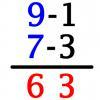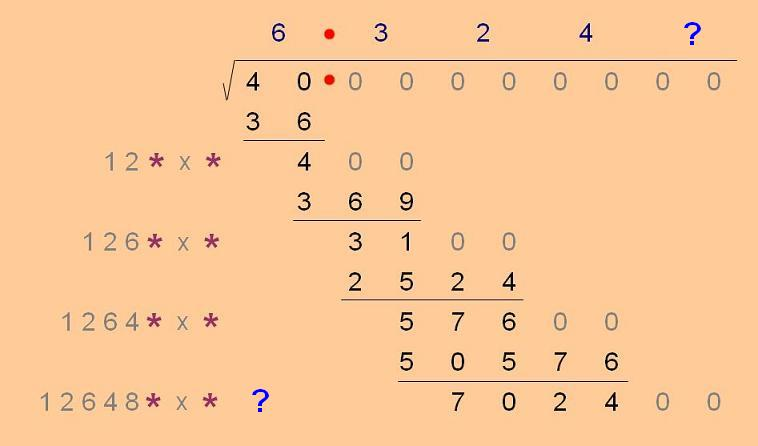#### You may also like### Triangle Incircle Iteration

Keep constructing triangles in the incircle of the previous triangle. What happens?### Vedic Sutra - All from 9 and Last from 10

Vedic Sutra is one of many ancient Indian sutras which involves a cross subtraction method. Can you give a good explanation of WHY it works?### Tournament Scheduling

Scheduling games is a little more challenging than one might desire. Here are some tournament formats that sport schedulers use.

# Unusual Long Division - Square Roots Before Calculators

##### Age 14 to 16Challenge Level

However did we manage before calculators? Let's think about that for a moment.

Just taking square roots as an example, every time we use Pythagoras to find the third side in a right-angled triangle we need to perform a square root. If it's the square root of a number like 4 that's easy : 2 . . plus or minus, if you like . . . but what if we needed the square root of 40?

Obviously it isn't 20, but what is it?

Now we do have a technique, one that we use in school from time to time, usually called 'trial and improvement', and it works like this: get close to the right answer, and then try to get closer still, being as systematic as possible, and continuing until we are close enough for our purposes.

Well that's good, it's nice to have a solution method, but before there were electronic calculators 'trial and improvement' could involve quite a lot of work, so people were motivated to find methods that used as little effort as possible.

Here's one of those methods, and your task is to decide how and why it works.Finding the square root of 40:

The answer is 6.324 . . . taking the method as far as three decimal places.

Notice the position of the decimal point and step away from there in both directions, two digits at a time. Each of those pairs will lead to one digit of the answer, and remember the zeros continue indefinitely.

Start on the left. The first pair is 40. Find the largest square smaller than 40, that's 36. Subtract the 36 from the 40, which leaves 4, and enter a 6 as the first digit of your answer.

Next take the 4 and 'bring down' the next pair of digits (00 as it happens) to make 400. We are trying to decide the second digit of our answer and we find it like this:

Use the 6 but double it (12) and make that ten times bigger (120). Now find 'something'- a single digit, so that one hundred and twenty 'something' times that same 'something' is as large as possible but less than the 400.

123 times 3 makes 369, so three is the digit we want (124 times 4 would have been too big).

Subtract the 369 from the 400 (31) and 'bring down' the next pair of digits (so we are now aiming for 3100).
The digits we have so far in the answer are 6 and 3. Double 63 and then make it ten times bigger (1260). Use the same technique as before: find one thousand two hundred and sixty 'something', times 'something', that gets as close as possible to 3100 without exceeding it.

Our third digit will be 2, 1262 times 2 is 2524, 1263 times 3 would be too large.

Subtract to leave 576, bring down the next pair of digits, double the digits you already have, that's 632, which doubles to make 1264, now look for twelve thousand, six hundred and forty 'something' times 'something' to come as close as possible to 57,600 without exceeding it. That 'something' is 4 (check it), and so we continue until we have as many digits in our answer as we think we need.

Your task is to find the next digit (the answer is on the Hint page), check you can repeat the method for other square roots (use your calculator to check your answers). Try to account for the method and explain why it works?